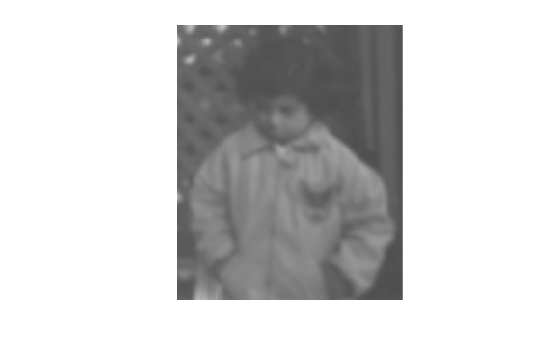Documentation

## Blur an Image Using an Average Filter

Read and display the input image.

``` I = imread('pout.tif'); imshow(I);```Compute the integral image.

` intImage = integralImage(I);`

Apply a 7-by-7 average filter.

``` avgH = integralKernel([1 1 7 7], 1/49); J = integralFilter(intImage, avgH);```

Cast the result back to the same class as the input image.

``` J = uint8(J); figure imshow(J);```Download ebook